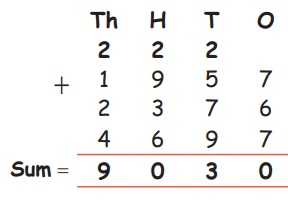Home | | Maths 4th Std | Adding 4 digit Numbers with regrouping

# Adding 4 digit Numbers with regrouping

Adding 4 digit Numbers with regrouping

Adding 4 digit Numbers with regrouping

(sum should not exceed 10000)

EXAMPLE

1. Add: Find the sum of 1957, 2376 and 4697Activity

Write the missing numbersTags : Numbers | Term 1 Chapter 2 | 4th Maths , 4th Maths : Term 1 Unit 2 : Numbers
Study Material, Lecturing Notes, Assignment, Reference, Wiki description explanation, brief detail
4th Maths : Term 1 Unit 2 : Numbers : Adding 4 digit Numbers with regrouping | Numbers | Term 1 Chapter 2 | 4th Maths